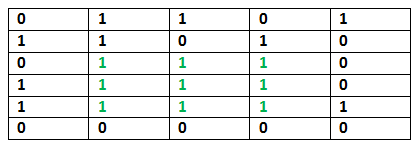# Java Program for Maximum size square sub-matrix with all 1s

Given a binary matrix, find out the maximum size square sub-matrix with all 1s.

For example, consider the below binary matrix.## Java

 `// JAVA Code for Maximum size square ` `// sub-matrix with all 1s ` `public` `class` `GFG { ` `    ``// method for Maximum size square sub-matrix with all 1s ` `    ``static` `void` `printMaxSubSquare(``int` `M[][]) ` `    ``{ ` `        ``int` `i, j; ` `        ``int` `R = M.length; ``// no of rows in M[][] ` `        ``int` `C = M[``0``].length; ``// no of columns in M[][] ` `        ``int` `S[][] = ``new` `int``[R][C]; ` ` `  `        ``int` `max_of_s, max_i, max_j; ` ` `  `        ``/* Set first column of S[][]*/` `        ``for` `(i = ``0``; i < R; i++) ` `            ``S[i][``0``] = M[i][``0``]; ` ` `  `        ``/* Set first row of S[][]*/` `        ``for` `(j = ``0``; j < C; j++) ` `            ``S[``0``][j] = M[``0``][j]; ` ` `  `        ``/* Construct other entries of S[][]*/` `        ``for` `(i = ``1``; i < R; i++) { ` `            ``for` `(j = ``1``; j < C; j++) { ` `                ``if` `(M[i][j] == ``1``) ` `                    ``S[i][j] = Math.min(S[i][j - ``1``], ` `                                       ``Math.min(S[i - ``1``][j], S[i - ``1``][j - ``1``])) ` `                              ``+ ``1``; ` `                ``else` `                    ``S[i][j] = ``0``; ` `            ``} ` `        ``} ` ` `  `        ``/* Find the maximum entry, and indexes of maximum entry  ` `            ``in S[][] */` `        ``max_of_s = S[``0``][``0``]; ` `        ``max_i = ``0``; ` `        ``max_j = ``0``; ` `        ``for` `(i = ``0``; i < R; i++) { ` `            ``for` `(j = ``0``; j < C; j++) { ` `                ``if` `(max_of_s < S[i][j]) { ` `                    ``max_of_s = S[i][j]; ` `                    ``max_i = i; ` `                    ``max_j = j; ` `                ``} ` `            ``} ` `        ``} ` ` `  `        ``System.out.println(``"Maximum size sub-matrix is: "``); ` `        ``for` `(i = max_i; i > max_i - max_of_s; i--) { ` `            ``for` `(j = max_j; j > max_j - max_of_s; j--) { ` `                ``System.out.print(M[i][j] + ``" "``); ` `            ``} ` `            ``System.out.println(); ` `        ``} ` `    ``} ` ` `  `    ``// Driver program ` `    ``public` `static` `void` `main(String[] args) ` `    ``{ ` `        ``int` `M[][] = { { ``0``, ``1``, ``1``, ``0``, ``1` `}, ` `                      ``{ ``1``, ``1``, ``0``, ``1``, ``0` `}, ` `                      ``{ ``0``, ``1``, ``1``, ``1``, ``0` `}, ` `                      ``{ ``1``, ``1``, ``1``, ``1``, ``0` `}, ` `                      ``{ ``1``, ``1``, ``1``, ``1``, ``1` `}, ` `                      ``{ ``0``, ``0``, ``0``, ``0``, ``0` `} }; ` ` `  `        ``printMaxSubSquare(M); ` `    ``} ` `} `

Output:

```Maximum size sub-matrix is:
1 1 1
1 1 1
1 1 1
```

Please refer complete article on Maximum size square sub-matrix with all 1s for more details!

My Personal Notes arrow_drop_up
Article Tags :

Be the First to upvote.

Please write to us at contribute@geeksforgeeks.org to report any issue with the above content.